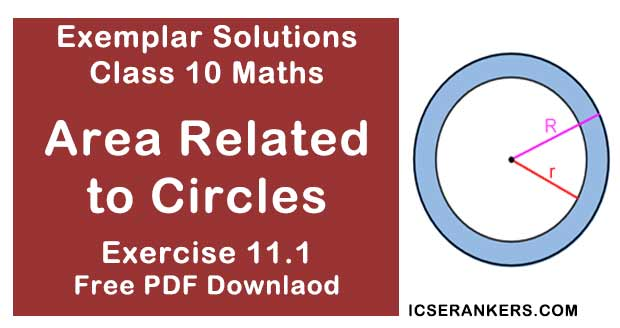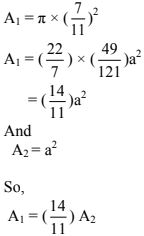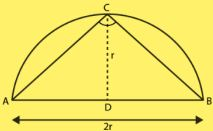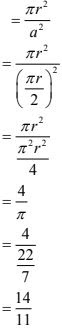# Chapter 11 Area Related to Circles NCERT Exemplar Solutions Exercise 11.1 Class 10 MathsChapter Name NCERT Maths Exemplar Solutions for Chapter 11 Area Related to Circles Exercise 11.1 Book Name NCERT Exemplar for Class 10 Maths Other Exercises Exercise 11.2Exercise 11.3Exercise 11.4 Related Study NCERT Solutions for Class 10 Maths

### Exercise 11.1 Solutions

Multiple Choice Questions

Choose the correct answer from the given four options:

1. If the sum of the areas of two circles with radii R1 and R2 is equal to the area of a circle of radius R, then:
(A) R1 + R2 = R
(B) R12 + R22 = R2
(C) R1 + R2 < R
(D) R12 + R22 < R2
Solution
(B) R12 + R22 = R2
Justification :
We have,
Area of circle  = Area of first circle + Area of second circle
πR2 = πR12 + πR22
⇒ R2 = R12 + R22
Option B is correct.

2. If the sum of the circumferences of two circles with radii R1 and R2 is equal to the circumference of a circle of radius R, then:
(A) R1 + R2 = R
(B) R1 + R2 > R
(C) R1 + R2 < R
(D) Nothing definite can be said about the relation among R1 , R2 & R.
Solution
(A) R1 + R2 = R
Justification :
We have,
Circumference of circle with radius R = Circumference of first circle with radius R1 +
Circumference of second circle with radius R2
πR = πR1 + πR2
⇒ R = R1 + R2
Option A is correct.

3. If the circumference of a circle and the perimeter of a square are equal, then:
(A) Area of the circle = Area of the square
(B) Area of the circle > Area of the square
(C) Area of the circle < Area of the square
(D) Nothing definite can be said about the relation between the areas of the circle & square.
Solution
(B) Area of the circle > Area of the square
Justification:
We have,
Circumference of a circle = Perimeter of square
Let r be the radius of the circle and a be the side of square.
From the given condition,
2πr = 4a
⇒ 11r = 7a
⇒ a = (11/7)r
⇒ r = (7/11)a
Now,
Area of circle = A1 = πr2 and
Area of square = A2 = a2
From equation (i), we haveWe get,
A1 > A2
Therefore, Area of the circle > Area of the square.
Option B is correct.

4. Area of the largest triangle that can be inscribed in a semi-circle of radius r units is
(A) r2 sq. units
(B) (1/2)r2 sq. units
(C) 2r2 sq. units
(D) √2r2 sq. units
Solution
(A) r2 sq. units
Justification:
The largest triangle that can be inscribed in a semi-circle of radius r units is the triangle having its base as the diameter of the semi-circle and the two other sides are taken by considering a point C on the circumference of the semi-circle and joining it by the end points of diameter A and B.∠ C = 90° (by the properties of circle)
ΔABC is right angled triangle with base as diameter AB of the circle and height be CD.
Height of the triangle = r
Area of largest ΔABC = (1/2) × Base × Height
= (1/2) × AB × CD
= (1/2) × 2r × r
= r2  sq. units
Option A is correct.

5. If the perimeter of a circle is equal to that of a square, then the ratio of their areas is
(A) 22 : 7
(B) 14 : 11
(C) 7 : 22
(D) 11: 14
Solution
(B) 14 : 11
Justification:
Let r be the radius of the circle and a be the side of the square.
We have,
Perimeter of a circle = Perimeter of a square
2πr = 4a
⇒ a = πr/2
Area of the circle = πr2 and
Area of the square = a2
Now,
Ratio of their areas = (Area of circle)/(Area of square)Option B is correct.

6. It is proposed to build a single circular park equal in area to the sum of areas of two circular parks of diameters 16 m and 12 m in a locality. The radius of the new park would be
(A) 10 m
(B) 15 m
(C) 20 m
(D) 24 m
Solution
(A) 10 m
We have,
Area of first Circular Park, whose diameter is 16 m,
= πr2/2
= π(16/2)2
= 64π sq. metre
Area of second Circular Park, whose diameter is 12m,
= πr2/2
= π(12/2)2
= 36π sq. metre
According to the question,
Area of single circular park = area of 1st circular park + area of 2nd circular park
πR2 = 36π + 64π
⇒ R2 = 100
⇒ R = 10 m

7. The area of the circle that can be inscribed in a square of side 6 cm is
(A) 36 π cm2
(B) 18π cm2
(C) 12π cm2
(D) 9π cm2
Solution
(D) 9π cm2
We have,
Side of square = 6cm
Side of square = diameter of circle = 6cm
So,
Radius of the circle = 3cm
Area of circle = πr2
= π32
= 9π cm2

8. The area of the square that can be inscribed in a circle of radius 8 cm is
(A) 256 cm2
(B) 128 cm2
(C) 64√2 cm2
(D) 64 cm2
Solution
(B) 128 cm2
We have,
Radius of circle, r = OC = 8cm.
Diameter of the circle = AC
= 2 × OC
= 2 × 8
= 16 cm
Which is equal to the diagonal of a square.
As square is inscribed in circle,
Diameter of the circle = Diagonal of a square
So,
Area of square,
= (Diagonal)2/2
= 162/2
= 256/2
= 128 cm2

9. The radius of a circle whose circumference is equal to the sum of the circumferences of the two circles of diameters 36cm and 20 cm is
(A) 56 cm
(B) 42 cm
(C) 28 cm
(D) 16 cm
Solution
(C) 28 cm
Circumference of first circle  = 2πr
= πd1
= 36π cm  [given, d1 = 36 cm]
Circumference of second circle = πd2
= 20π  cm  [given, d2 = 20 cm]
We have,
Circumference of circle  = Circumference of first circle + Circumference of second circle
πD = 36π + 20π
⇒ D = 56 cm
Required radius of circle = 28 cm

10. The diameter of a circle whose area is equal to the sum of the areas of the two circles of radii 24 cm and 7 cm is
(A) 31 cm
(B) 25 cm
(C) 62 cm
(D) 50 cm
Solution
(D) 50 cm
Let,
r1 = 24 cm
r2 = 7 cm
Area of first circle = πr12
= π(24)2
= 576 π cm2
Area of second circle = πr22
= π(7)2
= 49 π cm2
We have,
Area of circle = Area of first circle + Area of second circle
πR2 = 576π + 49π
⇒ R = 25 cm
Required diameter of circle = 2 × 25 = 50 cm